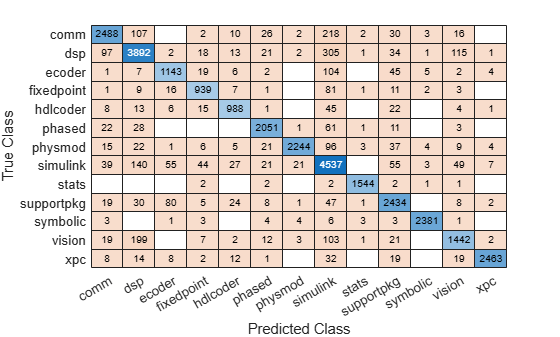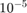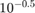# kfoldPredict

Predict labels for observations not used for training

## Syntax

``Label = kfoldPredict(CVMdl)``
``Label = kfoldPredict(CVMdl,Name,Value)``
``````[Label,NegLoss,PBScore] = kfoldPredict(___)``````
``````[Label,NegLoss,PBScore,Posterior] = kfoldPredict(___)``````

## Description

example

````Label = kfoldPredict(CVMdl)` returns class labels predicted by the cross-validated ECOC model composed of linear classification models `CVMdl`. That is, for every fold, `kfoldPredict` predicts class labels for observations that it holds out when it trains using all other observations. `kfoldPredict` applies the same data used create `CVMdl` (see `fitcecoc`).Also, `Label` contains class labels for each regularization strength in the linear classification models that compose `CVMdl`.```

example

````Label = kfoldPredict(CVMdl,Name,Value)` returns predicted class labels with additional options specified by one or more `Name,Value` pair arguments. For example, specify the posterior probability estimation method, decoding scheme, or verbosity level.```

example

``````[Label,NegLoss,PBScore] = kfoldPredict(___)``` additionally returns, for held-out observations and each regularization strength: Negated values of the average binary loss per class (`NegLoss`).Positive-class scores (`PBScore`) for each binary learner. ```

example

``````[Label,NegLoss,PBScore,Posterior] = kfoldPredict(___)``` additionally returns posterior class probability estimates for held-out observations and for each regularization strength. To return posterior probabilities, the linear classification model learners must be logistic regression models.```

## Input Arguments

expand all

Cross-validated, ECOC model composed of linear classification models, specified as a `ClassificationPartitionedLinearECOC` model object. You can create a `ClassificationPartitionedLinearECOC` model using `fitcecoc` and by:

1. Specifying any one of the cross-validation, name-value pair arguments, for example, `CrossVal`

2. Setting the name-value pair argument `Learners` to `'linear'` or a linear classification model template returned by `templateLinear`

To obtain estimates, kfoldPredict applies the same data used to cross-validate the ECOC model (`X` and `Y`).

### Name-Value Arguments

Specify optional pairs of arguments as `Name1=Value1,...,NameN=ValueN`, where `Name` is the argument name and `Value` is the corresponding value. Name-value arguments must appear after other arguments, but the order of the pairs does not matter.

Before R2021a, use commas to separate each name and value, and enclose `Name` in quotes.

Binary learner loss function, specified as the comma-separated pair consisting of `'BinaryLoss'` and a built-in loss function name or function handle.

• This table contains names and descriptions of the built-in functions, where yj is the class label for a particular binary learner (in the set {-1,1,0}), sj is the score for observation j, and g(yj,sj) is the binary loss formula.

ValueDescriptionScore Domaing(yj,sj)
`'binodeviance'`Binomial deviance(–∞,∞)log[1 + exp(–2yjsj)]/[2log(2)]
`'exponential'`Exponential(–∞,∞)exp(–yjsj)/2
`'hamming'`Hamming[0,1] or (–∞,∞)[1 – sign(yjsj)]/2
`'hinge'`Hinge(–∞,∞)max(0,1 – yjsj)/2
`'linear'`Linear(–∞,∞)(1 – yjsj)/2
`'logit'`Logistic(–∞,∞)log[1 + exp(–yjsj)]/[2log(2)]
`'quadratic'`Quadratic[0,1][1 – yj(2sj – 1)]2/2

The software normalizes the binary losses such that the loss is 0.5 when yj = 0. Also, the software calculates the mean binary loss for each class.

• For a custom binary loss function, e.g., `customFunction`, specify its function handle `'BinaryLoss',@customFunction`.

`customFunction` should have this form

`bLoss = customFunction(M,s)`
where:

• `M` is the K-by-B coding matrix stored in `Mdl.CodingMatrix`.

• `s` is the 1-by-B row vector of classification scores.

• `bLoss` is the classification loss. This scalar aggregates the binary losses for every learner in a particular class. For example, you can use the mean binary loss to aggregate the loss over the learners for each class.

• K is the number of classes.

• B is the number of binary learners.

For an example of passing a custom binary loss function, see Predict Test-Sample Labels of ECOC Model Using Custom Binary Loss Function.

By default, if all binary learners are linear classification models using:

• SVM, then `BinaryLoss` is `'hinge'`

• Logistic regression, then `BinaryLoss` is `'quadratic'`

Example: `'BinaryLoss','binodeviance'`

Data Types: `char` | `string` | `function_handle`

Decoding scheme that aggregates the binary losses, specified as the comma-separated pair consisting of `'Decoding'` and `'lossweighted'` or `'lossbased'`. For more information, see Binary Loss.

Example: `'Decoding','lossbased'`

Number of random initial values for fitting posterior probabilities by Kullback-Leibler divergence minimization, specified as the comma-separated pair consisting of `'NumKLInitializations'` and a nonnegative integer.

To use this option, you must:

• Return the fourth output argument (`Posterior`).

• The linear classification models that compose the ECOC models must use logistic regression learners (that is, `CVMdl.Trained{1}.BinaryLearners{1}.Learner` must be `'logistic'`).

• `PosteriorMethod` must be `'kl'`.

For more details, see Posterior Estimation Using Kullback-Leibler Divergence.

Example: `'NumKLInitializations',5`

Data Types: `single` | `double`

Estimation options, specified as the comma-separated pair consisting of `'Options'` and a structure array returned by `statset`.

To invoke parallel computing:

• You need a Parallel Computing Toolbox™ license.

• Specify `'Options',statset('UseParallel',true)`.

Posterior probability estimation method, specified as the comma-separated pair consisting of `'PosteriorMethod'` and `'kl'` or `'qp'`.

• To use this option, you must return the fourth output argument (`Posterior`) and the linear classification models that compose the ECOC models must use logistic regression learners (that is, `CVMdl.Trained{1}.BinaryLearners{1}.Learner` must be `'logistic'`).

• If `PosteriorMethod` is `'kl'`, then the software estimates multiclass posterior probabilities by minimizing the Kullback-Leibler divergence between the predicted and expected posterior probabilities returned by binary learners. For details, see Posterior Estimation Using Kullback-Leibler Divergence.

• If `PosteriorMethod` is `'qp'`, then the software estimates multiclass posterior probabilities by solving a least-squares problem using quadratic programming. You need an Optimization Toolbox™ license to use this option. For details, see Posterior Estimation Using Quadratic Programming.

Example: `'PosteriorMethod','qp'`

Verbosity level, specified as the comma-separated pair consisting of `'Verbose'` and `0` or `1`. `Verbose` controls the number of diagnostic messages that the software displays in the Command Window.

If `Verbose` is `0`, then the software does not display diagnostic messages. Otherwise, the software displays diagnostic messages.

Example: `'Verbose',1`

Data Types: `single` | `double`

## Output Arguments

expand all

Cross-validated, predicted class labels, returned as a categorical or character array, logical or numeric matrix, or cell array of character vectors.

In most cases, `Label` is an n-by-L array of the same data type as the observed class labels (`Y`) used to create `CVMdl`. (The software treats string arrays as cell arrays of character vectors.) n is the number of observations in the predictor data (`X`) and L is the number of regularization strengths in the linear classification models that compose the cross-validated ECOC model. That is, `Label(i,j)` is the predicted class label for observation `i` using the ECOC model of linear classification models that has regularization strength `CVMdl.Trained{1}.BinaryLearners{1}.Lambda(j)`.

If `Y` is a character array and L > 1, then `Label` is a cell array of class labels.

The software assigns the predicted label corresponding to the class with the largest, negated, average binary loss (`NegLoss`), or, equivalently, the smallest average binary loss.

Cross-validated, negated, average binary losses, returned as an n-by-K-by-L numeric matrix or array. K is the number of distinct classes in the training data and columns correspond to the classes in `CVMdl.ClassNames`. For n and L, see `Label`. `NegLoss(i,k,j)` is the negated, average binary loss for classifying observation `i` into class `k` using the linear classification model that has regularization strength `CVMdl.Trained{1}.BinaryLoss{1}.Lambda(j)`.

• If `Decoding` is `'lossbased'`, then `NegLoss(i,k,j)` is the sum of the binary losses divided by the total number of binary learners.

• If `Decoding` is `'lossweighted'`, then `NegLoss(i,k,j)` is the sum of the binary losses divided by the number of binary learners for the kth class.

For more details, see Binary Loss.

Cross-validated, positive-class scores, returned as an n-by-B-by-L numeric array. B is the number of binary learners in the cross-validated ECOC model and columns correspond to the binary learners in `CVMdl.Trained{1}.BinaryLearners`. For n and L, see `Label`. `PBScore(i,b,j)` is the positive-class score of binary learner b for classifying observation `i` into its positive class, using the linear classification model that has regularization strength `CVMdl.Trained{1}.BinaryLearners{1}.Lambda(j)`.

If the coding matrix varies across folds (that is, if the coding scheme is `sparserandom` or `denserandom`), then `PBScore` is empty (`[]`).

Cross-validated posterior class probabilities, returned as an n-by-K-by-L numeric array. For dimension definitions, see `NegLoss`. `Posterior(i,k,j)` is the posterior probability for classifying observation `i` into class `k` using the linear classification model that has regularization strength `CVMdl.Trained{1}.BinaryLearners{1}.Lambda(j)`.

To return posterior probabilities, `CVMdl.Trained{1}.BinaryLearner{1}.Learner` must be `'logistic'`.

## Examples

expand all

`load nlpdata`

`X` is a sparse matrix of predictor data, and `Y` is a categorical vector of class labels.

Cross-validate an ECOC model of linear classification models.

```rng(1); % For reproducibility CVMdl = fitcecoc(X,Y,'Learner','linear','CrossVal','on');```

`CVMdl` is a `ClassificationPartitionedLinearECOC` model. By default, the software implements 10-fold cross validation.

Predict labels for the observations that `fitcecoc` did not use in training the folds.

`label = kfoldPredict(CVMdl);`

Because there is one regularization strength in `CVMdl`, `label` is a column vector of predictions containing as many rows as observations in `X`.

Construct a confusion matrix.

`cm = confusionchart(Y,label);`Load the NLP data set. Transpose the predictor data.

```load nlpdata X = X';```

For simplicity, use the label 'others' for all observations in `Y` that are not `'simulink'`, `'dsp'`, or `'comm'`.

`Y(~(ismember(Y,{'simulink','dsp','comm'}))) = 'others';`

Create a linear classification model template that specifies optimizing the objective function using SpaRSA.

`t = templateLinear('Solver','sparsa');`

Cross-validate an ECOC model of linear classification models using 5-fold cross-validation. Specify that the predictor observations correspond to columns.

```rng(1); % For reproducibility CVMdl = fitcecoc(X,Y,'Learners',t,'KFold',5,'ObservationsIn','columns'); CMdl1 = CVMdl.Trained{1}```
```CMdl1 = CompactClassificationECOC ResponseName: 'Y' ClassNames: [comm dsp simulink others] ScoreTransform: 'none' BinaryLearners: {6x1 cell} CodingMatrix: [4x6 double] ```

`CVMdl` is a `ClassificationPartitionedLinearECOC` model. It contains the property `Trained`, which is a 5-by-1 cell array holding a `CompactClassificationECOC` models that the software trained using the training set of each fold.

By default, the linear classification models that compose the ECOC models use SVMs. SVM scores are signed distances from the observation to the decision boundary. Therefore, the domain is $\left(-\infty ,\infty \right)$. Create a custom binary loss function that:

• Maps the coding design matrix (M) and positive-class classification scores (s) for each learner to the binary loss for each observation

• Uses linear loss

• Aggregates the binary learner loss using the median.

You can create a separate function for the binary loss function, and then save it on the MATLAB® path. Or, you can specify an anonymous binary loss function.

`customBL = @(M,s)median(1 - (M.*s),2,'omitnan')/2;`

Predict cross-validation labels and estimate the median binary loss per class. Print the median negative binary losses per class for a random set of 10 out-of-fold observations.

```[label,NegLoss] = kfoldPredict(CVMdl,'BinaryLoss',customBL); idx = randsample(numel(label),10); table(Y(idx),label(idx),NegLoss(idx,1),NegLoss(idx,2),NegLoss(idx,3),... NegLoss(idx,4),'VariableNames',[{'True'};{'Predicted'};... categories(CVMdl.ClassNames)])```
```ans=10×6 table True Predicted comm dsp simulink others ________ _________ _________ ________ ________ _______ others others -1.2319 -1.0488 0.048758 1.6175 simulink simulink -16.407 -12.218 21.531 11.218 dsp dsp -0.7387 -0.11534 -0.88466 -0.2613 others others -0.1251 -0.8749 -0.99766 0.14517 dsp dsp 2.5867 6.4187 -3.5867 -4.4165 others others -0.025358 -1.2287 -0.97464 0.19747 others others -2.6725 -0.56708 -0.51092 2.7453 others others -1.1605 -0.88321 -0.11679 0.43504 others others -1.9511 -1.3175 0.24735 0.95111 simulink others -7.848 -5.8203 4.8203 6.8457 ```

The software predicts the label based on the maximum negated loss.

ECOC models composed of linear classification models return posterior probabilities for logistic regression learners only. This example requires the Parallel Computing Toolbox™ and the Optimization Toolbox™

Load the NLP data set and preprocess the data as in Specify Custom Binary Loss.

```load nlpdata X = X'; Y(~(ismember(Y,{'simulink','dsp','comm'}))) = 'others'; ```

Create a set of 5 logarithmically-spaced regularization strengths fromthrough.

```Lambda = logspace(-6,-0.5,5); ```

Create a linear classification model template that specifies optimizing the objective function using SpaRSA and to use logistic regression learners.

```t = templateLinear('Solver','sparsa','Learner','logistic','Lambda',Lambda); ```

Cross-validate an ECOC model of linear classification models using 5-fold cross-validation. Specify that the predictor observations correspond to columns, and to use parallel computing.

```rng(1); % For reproducibility Options = statset('UseParallel',true); CVMdl = fitcecoc(X,Y,'Learners',t,'KFold',5,'ObservationsIn','columns',... 'Options',Options); ```
```Starting parallel pool (parpool) using the 'local' profile ... Connected to the parallel pool (number of workers: 6). ```

Predict the cross-validated posterior class probabilities. Specify to use parallel computing and to estimate posterior probabilities using quadratic programming.

```[label,~,~,Posterior] = kfoldPredict(CVMdl,'Options',Options,... 'PosteriorMethod','qp'); size(label) label(3,4) size(Posterior) Posterior(3,:,4) ```
```ans = 31572 5 ans = categorical others ans = 31572 4 5 ans = 0.0285 0.0373 0.1714 0.7627 ```

Because there are five regularization strengths:

• `label` is a 31572-by-5 categorical array. `label(3,4)` is the predicted, cross-validated label for observation 3 using the model trained with regularization strength `Lambda(4)`.

• `Posterior` is a 31572-by-4-by-5 matrix. `Posterior(3,:,4)` is the vector of all estimated, posterior class probabilities for observation 3 using the model trained with regularization strength `Lambda(4)`. The order of the second dimension corresponds to `CVMdl.ClassNames`. Display a random set of 10 posterior class probabilities.

Display a random sample of cross-validated labels and posterior probabilities for the model trained using `Lambda(4)`.

```idx = randsample(size(label,1),10); table(Y(idx),label(idx,4),Posterior(idx,1,4),Posterior(idx,2,4),... Posterior(idx,3,4),Posterior(idx,4,4),... 'VariableNames',[{'True'};{'Predicted'};categories(CVMdl.ClassNames)]) ```
```ans = 10×6 table True Predicted comm dsp simulink others ________ _________ __________ __________ ________ _________ others others 0.030275 0.022142 0.10416 0.84342 simulink simulink 3.4954e-05 4.2982e-05 0.99832 0.0016016 dsp others 0.15787 0.25718 0.18848 0.39647 others others 0.094177 0.062712 0.12921 0.71391 dsp dsp 0.0057979 0.89703 0.015098 0.082072 others others 0.086084 0.054836 0.086165 0.77292 others others 0.0062338 0.0060492 0.023816 0.9639 others others 0.06543 0.075097 0.17136 0.68812 others others 0.051843 0.025566 0.13299 0.7896 simulink simulink 0.00044059 0.00049753 0.70958 0.28948 ```

expand all

## Algorithms

expand all

The software can estimate class posterior probabilities by minimizing the Kullback-Leibler divergence or by using quadratic programming. For the following descriptions of the posterior estimation algorithms, assume that:

• mkj is the element (k,j) of the coding design matrix M.

• I is the indicator function.

• ${\stackrel{^}{p}}_{k}$ is the class posterior probability estimate for class k of an observation, k = 1,...,K.

• rj is the positive-class posterior probability for binary learner j. That is, rj is the probability that binary learner j classifies an observation into the positive class, given the training data.

 Allwein, E., R. Schapire, and Y. Singer. “Reducing multiclass to binary: A unifying approach for margin classiﬁers.” Journal of Machine Learning Research. Vol. 1, 2000, pp. 113–141.

 Dietterich, T., and G. Bakiri. “Solving Multiclass Learning Problems Via Error-Correcting Output Codes.” Journal of Artificial Intelligence Research. Vol. 2, 1995, pp. 263–286.

 Escalera, S., O. Pujol, and P. Radeva. “Separability of ternary codes for sparse designs of error-correcting output codes.” Pattern Recog. Lett., Vol. 30, Issue 3, 2009, pp. 285–297.

 Escalera, S., O. Pujol, and P. Radeva. “On the decoding process in ternary error-correcting output codes.” IEEE Transactions on Pattern Analysis and Machine Intelligence. Vol. 32, Issue 7, 2010, pp. 120–134.

 Hastie, T., and R. Tibshirani. “Classification by Pairwise Coupling.” Annals of Statistics. Vol. 26, Issue 2, 1998, pp. 451–471.

 Wu, T. F., C. J. Lin, and R. Weng. “Probability Estimates for Multi-Class Classification by Pairwise Coupling.” Journal of Machine Learning Research. Vol. 5, 2004, pp. 975–1005.

 Zadrozny, B. “Reducing Multiclass to Binary by Coupling Probability Estimates.” NIPS 2001: Proceedings of Advances in Neural Information Processing Systems 14, 2001, pp. 1041–1048.